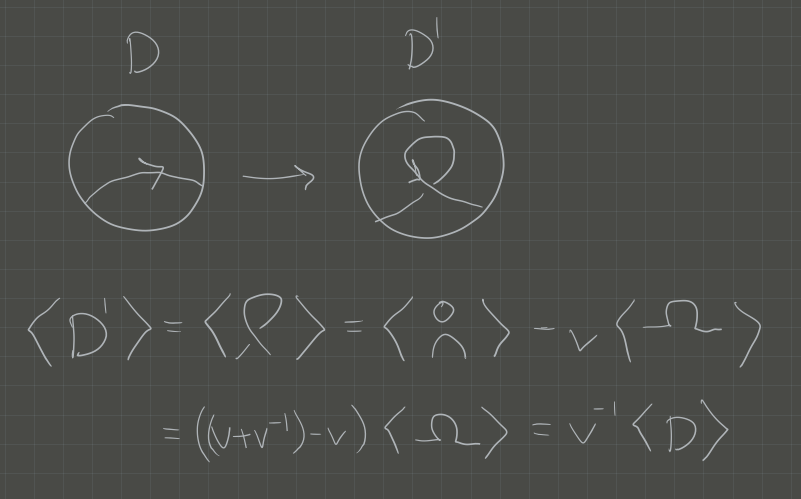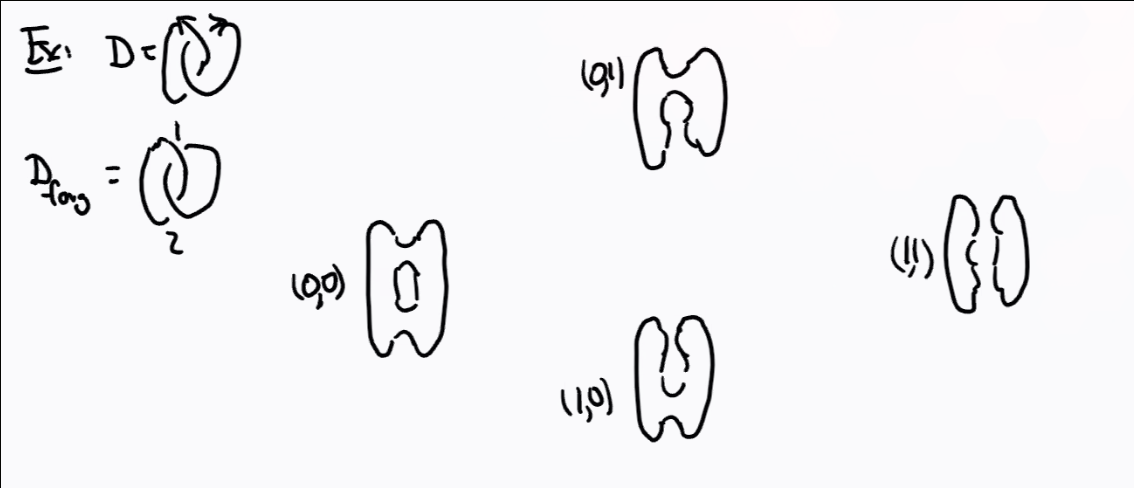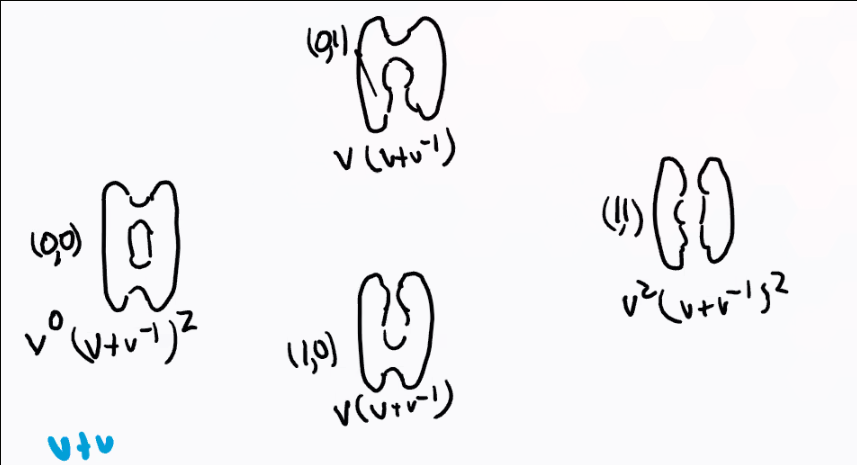# Monday July 6th

## Motivation

We’ll start with $$X$$ a finite CW complex.

A CW complex is a topological space built by inductively attaching $$i{\hbox{-}}$$dimensional discs ($$i{\hbox{-}}$$cells) $${\mathbb{D}}^i \mathrel{\vcenter{:}}=\left\{{\mathbf{x}\in {\mathbb{R}}^i {~\mathrel{\Big|}~}{\left\lVert {\mathbf{x}} \right\rVert} \leq 1}\right\}$$ along their boundary $${{\partial}}{\mathbb{D}}^i = S^{i-1} \mathrel{\vcenter{:}}=\left\{{\mathbf{x}\in {\mathbb{R}}^i {~\mathrel{\Big|}~}{\left\lVert {\mathbf{x}} \right\rVert} = 1}\right\}$$.

Define $$\chi(X) = \sum_{i\in {\mathbb{Z}}} (-1)^i {\left\lvert {C_i} \right\rvert}$$ where $${\left\lvert {C_i} \right\rvert}$$ is the number of $$i{\hbox{-}}$$cells.

Note that a homotopy equivalence between spaces induces an equality between Euler characteristics.

Recall that we can define the cellular chain complex \begin{align*} C_*^{\text{cell}}(X, {\mathbb{C}})\mathrel{\vcenter{:}}=\cdots \xrightarrow{{\partial}_{i+1}} C_n^{\text{cell}} (X, {\mathbb{C}}) \xrightarrow{{\partial}_{i}} \cdots \to C_0^{\text{cell}}(X, {\mathbb{C}}) \end{align*}

and $$H_i(X, {\mathbb{C}}) \mathrel{\vcenter{:}}=\ker {\partial}_i / \operatorname{im}({\partial})_{i+1}$$.

\begin{align*} \sum (-1)^i \dim H_i(X, {\mathbb{C}}) = \chi(X) \end{align*}

In this sense, cellular homology categorifies the Euler characteristic: we’ve replaced a set of objects with a category. This is an improvement because we may not have maps between the elements of sets, but we do have maps between objects in a category. We can also talk about things such as functoriality.

The euler characteristic is a weaker invariant than homology. Note that \begin{align*} \chi(S^1) = 0 &\quad\text{and}\quad \chi(S^1{\coprod}S^1) = 0 \\ \\ H_0(S^1) = {\mathbb{C}}&\quad\text{while}\quad H_0(S^1{\coprod}S^1) = {\mathbb{C}}\oplus {\mathbb{C}} ,\end{align*} so these aren’t distinguished by euler characteristic alone.

Our first goal will be to assign invariants to oriented links $$L$$, where homotopy equivalence will be replaced with isotopy. We’ll assign a Khovanov complex $$C_*(L,{\mathbb{C}})$$, a complex of $${\mathbb{Z}}{\hbox{-}}$$graded $${\mathbb{C}}{\hbox{-}}$$vector spaces, along with the Jones polynomial $$J(L) \in {\mathbb{Z}}[t, t^{-1}]$$. By taking the (graded) Euler characteristic of the chain complex, we’ll recover $$J(L)$$.

## Setup

A link $$L$$ is a smooth, closed 1-dimensional embedded submanifold of $${\mathbb{R}}^3$$. $$L$$ is a knot if it consists of one connected component.

We have planar projections:

Under this correspondence, isotopy of knots will correspond to planar isotopy of the diagrams and the following 3 Reidemeister moves:

There are three planar moves that preserve the isotopy class of a planar projection of a knot:

How to change knot diagrams using Reidemeister moves:

We now want to take an oriented, planar link diagram $$D$$ and associate to it a polynomial $$J(D)$$. We start by defining the Kauffman bracket

Let $$D_f$$ be $$D$$ with the orientation forgotten, then $$\left\langle{D_f}\right\rangle \in {\mathbb{Z}}[v, v^{-1}]$$ is defined recursively by

In the last case, the first term is a “0-resolution/0-smoothing” and the second is a “1-resolution/1-smoothing”.

We have a notion of positive/negative crossings:

We set \begin{align*} J(D) = (-1)^{n_-} v^{n^+ - 2n_-} \left\langle{D_f}\right\rangle .\end{align*}

1. $$J(S^1) = v + v^{-1}$$

2. $$J(?) = (-1) v^{-2} \qty{ -v^2 (v+v^{-1})} = v+v^{-1}$$.

3. $$J(?) = v^{-6} + v^{-4} + v^{-2} + 1$$

The Jones polynomial is invariant under move $$R1$$.

Can be checked in diagrams:$$J(D') = J(D)$$

You can now check that \begin{align*} J(D') = (-1)^{n_-(D)} v^{n_+(D) + 1} - 2n_- .\end{align*}

Check invariance under R2, R3.

# Tuesday July 7th

Recall that we had recursive rules for computing the Kausffman bracket, and a normalization factor for the Jones polynomial that made it into an invariant. We’d like a closed formula for these.

We do this by ordering the crossings of the unoriented link $$1, \cdots, n$$, then there is a correspondence \begin{align*} \left\{{0, 1}\right\}^n &\iff \text{Complete resolutions} \\ (\alpha_1, \cdots, \alpha_n) &\iff \alpha_i \text{ resolves the ith crossing} .\end{align*}\begin{align*} \left\langle{D}\right\rangle = \sum_{\alpha \in \left\{{0, 1}\right\}^n} (-1)^{{\left\lvert {\alpha} \right\rvert}} v^{{\left\lvert {\alpha} \right\rvert}} (v+v^{-1})^{c_\alpha(D)} ,\end{align*}

where $${\left\lvert {\alpha} \right\rvert}$$ is the number of 1-resolutions and $$c_\alpha$$ is the number of circles in the resolution corresponding to $$\alpha$$.

Idea: look at resolving the $$n$$th crossing locally and apply the recursive relation. Then rewrite the sum by appending $$\alpha_n = 0$$ and $$\alpha_n = 1$$ respectively. Note that we can rewrite the sum as \begin{align*} \sum_{r=0}^n (-1)^r \sum_{{\left\lvert {\alpha } \right\rvert}= r} v^r (v+v^{-1})^{c_\alpha(D)} .\end{align*}

This amounts to summing over the “columns” in the previous diagram:Here this yields \begin{align*} (v+v^{-1})^2 + (-1)2v(v+v^{-1}) + v^2 (v+v^{-1})^2 .\end{align*}

Note that this formula starts to resemble an Euler characteristic!

Problem: The coefficient \begin{align*} \sum v^r(v+v^{-1})^{c_\alpha(D)} \in {\mathbb{Z}}^{\geq 0}[v, v^{-1}] \end{align*} is a Laurent polynomial instead of a natural number, so this can’t immediately be interpreted as a dimension of a vector space.

Solution: Replace finite-dimensional $${\mathbb{C}}{\hbox{-}}$$vector spaces by $${\mathbb{Z}}{\hbox{-}}$$graded vector spaces. The category consists of objects given by $$V = \bigoplus_{i\in {\mathbb{Z}}} V_i$$ and linear maps $$f:V\to W$$ such that $$f(V_i) \subseteq W_i$$ for all $$i$$.

We previously had vector spaces categorifying the natural numbers by taking the dimension, so for graded vector spaces, we take the graded dimension:

\begin{align*} {\operatorname{gr\,dim}}\bigoplus_{i\in{\mathbb{Z}}}V_i = \sum_{i\in {\mathbb{Z}}}\qty{\dim V_i}v^i \in {\mathbb{Z}}^{\geq 0}[v, v^{-1}] .\end{align*}

Goal: We want to associate to an oriented link diagram $$D$$ a cochain complex of finite-dimensional graded $${\mathbb{C}}{\hbox{-}}$$vector spaces $$C_i(D) \xrightarrow{{{\partial}}} C_{i+1}(D) \to \cdots$$. Since each chain space decomposes, the differential does as well, and we get a large collection of chain complexes